# Category Archives: LTE

A 4G technology that is now being replaced by 5G worldwide.

# Eclipse

#### Eclipse 1.0 – A Paradigm Shift in RF Planning

NEW: Simulation of a Moving Transmitter (such as a car)

NEW: Simulation of a Moving Transmitter (such as a pedestrian)

Radio frequency planning is an essential component of network planning, roll-out, up-gradation, expansion etc. Several methods can be adopted for this from something as simple as free space models, empirical path loss models to the significantly more complicated, time consuming and expensive drive testing. Drive testing gives very accurate results but these results can be rendered useless by changing the position of an antenna or the tilt or transmit power of an antenna requiring another run in the field. One solution to this problem is ray-tracing which is very accurate but is usually considered to be very computationally expensive and of little practical value. But recent advances in computational power of machines coupled with efficient techniques have given a new lease of life to this method.

Eclipse is a near real-time simulation software for prediction of signal strength in urban areas. The software uses shooting and bouncing ray (SBR) method of ray tracing with 1 degree ray separation, 1 m step size and 9 interactions per ray path. The simulation parameters can be varied according to the resolution required. The code is highly optimized to give results in shortest possible time. It is especially useful for network planning of ultra-dense wireless networks where a dense network of antennas is placed on lamp posts instead of telecom towers. Various frequency bands can be simulated, along with different antenna radiation patterns and MIMO configurations.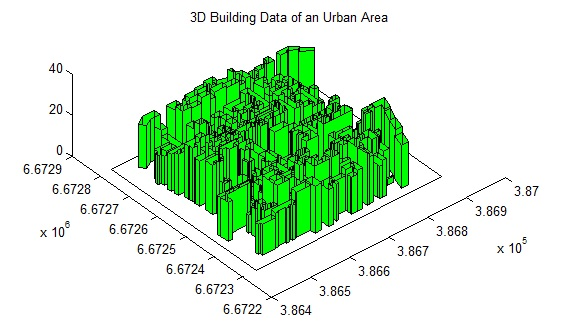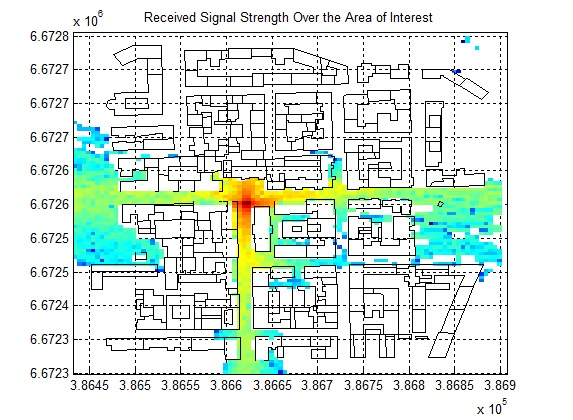Note: If you would like to run a test simulation send us a request at info@raymaps.com

In the previous posts we had discussed generation of a correlated Rayleigh fading sequence using Smith’s method  and Young’s modification of Smith’s method . The main contribution of Young was that he proposed a mechanism where the number of IDFTs was reduced by half. This was achieved by first adding two length N IID zero mean Gaussian sequences filtered by the filter F[k] and then performing the IDFT on the resulting complex sequence.

This was different to Smith’s method where the IDFT was performed simultaneously on two branches and then the outputs of these branches were added in quadrature to achieve the desired sequence with Rayleigh distributed envelope and Uniformly distributed phase. Another problem with Smith’s method was that the outputs of the two arms after performing IDFTs was assumed to be real which is not always the case in implementation and depends upon the combination of Doppler frequency (fm) and length of Gaussian sequence (N).

Young’s technique is shown graphically in the above figure. Also shown is the definition of filter F[k] which depends upon N, fm and km (please note that the fm in the above equation is normalized by the sampling frequency fs). Here km = N*(fm/fs). We propose three modifications to Young’s technique which significantly reduces computation and at the same time maintains the statistical properties of the generated sequence. The modifications we propose are.

1. First modification has to do with the generated Gaussian sequence. It is observed that the filter F[k], at very high sampling rates, is mostly zero and there are very few points which have some non-zero value. So when we multiply the Gaussian sequence with the filter we mostly get zeros at the output. So we propose that the filter response in the frequency domain must be calculated first and the the Gaussian random sequence must be generated for only those points where the filter F[k] is non-zero e.g. for a sampling frequency of 7.68 MHz (standard sampling frequency for a BW of 5 MHz in LTE) and Doppler frequency of 70 Hz (corresponding to medium Doppler case in LTE) the filter F[k] has 99.9982% zeros in its frequency response and it would be a highly wasteful to calculate Gaussian RVs for all those values.

2. Secondly according to Clarke  the Doppler Spectrum measurements have “Marked disagreement at very low frequencies and at frequencies in the region of the sharp cut-off associated with the maximum Doppler frequency shift. At very low frequencies the spectral energy is always observed to be higher than that predicted by theory”. He goes on to add “The reason for this is that neither theoretical model takes into account the large scale variations in total energy which result from the changing topography between transmitter and mobile receiver”. This suggests that the Classical Doppler Spectrum might not be the best choice under all scenarios. This has also been noted in  where a flat fading model is evaluated in terms of its Level Crossing Rate and Average Fade Duration. Such a flat spectrum is especially suited to indoor scenarios as noted in  and .

We propose a filter that gives equal weight to all the frequencies up to the maximum Doppler frequency. So our filter is a box-type filter which applies a constant scaling factor to all the frequencies in the pass-band and zeros out all the frequencies in the stop-band. So in fact the Gaussian sequence that is generated in the in-phase arm may directly be added with the Gaussian sequence from the other arm without applying the frequency domain filter and then IDFT of the complex sequence is taken. We will look into the deviation from ideality  that this causes later.

3. The third modification that we propose is in the implementation of the IDFT. Here again we take into consideration that the complex sequence being fed to the IDFT is filled with zeros (as we noted earlier 99.9982% zeros for 7.68 MHz and even more for higher frequencies) so we can avoid a lot multiplications and summations. The IDFT is defined below and also given is our modification to it.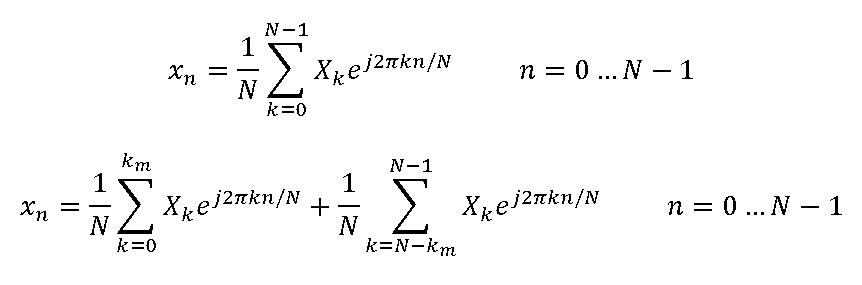Further improvement in computation time is achieved by implementing the above as a matrix multiplication. The matrix multiplication is implemented as H*X where H is the IDFT coefficients of size N x 2(km+1) and X is a vector of size 2(km+1) x 1 upon which the IDFT has to be performed.

Now let us look at the output sequence generated by using the above techniques. We consider the case of Medium Doppler Frequency of 70 Hz (EVA channel) as defined by LTE specifications. Sampling frequency is fixed at 10 kHz giving a normalized Doppler frequency of 0.007.  This was done due to limitation of memory on the machine. The author also experimented with a sampling frequency of 7.68 MHz but this did not yield enough samples for statistically accurate results. We did use a sampling frequency of 7.68 MHz for our bit error rate simulation which is shown in the end.

It is observed for fm=70 Hz the envelope of the output sequence using the proposed technique matches quite well with the envelope of the output sequence generated by the ideal filter proposed by Young. Also the phase and envelope of the sequence generated using the proposed technique has the desired distribution. Some of the other metrics that we can look at are the level crossing rate (LCR) and average fade duration (AFD) as well as the Auto Correlation of the real and imaginary parts of the complex sequence generated.

 Parameter Young Modified LCR (ideal) 48.1086 48.1086 LCR (sim) 48.1506 39.4348 AFD (ideal) 0.0018 0.0018 AFD (sim) 0.0018 0.0022

If we look at the results for LCR and AFD we see that the simulated results match reasonably well with the results predicted by theory. These results correspond to 100 snapshots of the fading sequence. It was important to take the average of several snapshots as results varied with each simulation run. Sometimes Young’s technique produced more accurate results while at other times the proposed technique was better. Again the limitations of computer memory and processing power dictated the length of the sequence that could be generated.

In general Young’s technique produced better results than our proposed technique. It was found that product of LCR and AFD for both cases matched quite well with the theoretical value. So the total time spent in a fade state per second was equal in both the cases. In the proposed method the duration of a single fading event was higher,  whereas the number of fading events per second was lower. This can be attributed to the fact that in our proposed technique higher weighting is given to lower frequency components and the fading sequence is smoothed out by these low frequency components. One technique to overcome this is spectral broadening as suggested by  but this is not the subject here and we postpone its discussion to another article.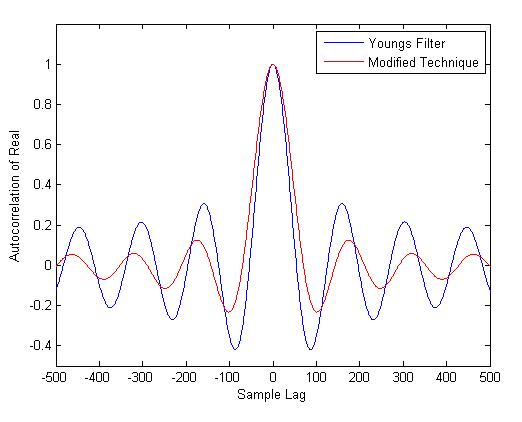Auto Correlation of Real Part fm=70Hz

The Auto Correlation of the real and imaginary parts of the generated sequences are also calculated for a Doppler frequency of 70 Hz. It is found that the Auto Correlation sequence for the two techniques matches quite well. However, the Auto Correlation sequence deviates from the theoretical value as calculated the by Bessel function of the first kind and zero order. Since we have used a flat spectral mask the Auto Correlation function resembles the sinc(x) function which is the same as zeroth order Spherical Bessel Function of the first kind (which is related to 1/2 order Bessel Function of the first kind).

It was found that when the Rayleigh fading sequence is generated by the program provided in Young’s thesis the shape of the Auto Correlation function depends upon the sampling frequency. At a normalized Doppler frequency of 0.05 and N=2^16 Young’s technique produces quite accurate results. We also measured the mean squared error (MSE) between the two Auto Correlations sequences and found it to be a function of the Normalized Doppler Frequency. It was found that as the Normalized Doppler Frequency was increased from 0.00007 to 0.0007 the MSE error dropped from 0.0277 to 0.0041. The relationship between the Normalized Doppler Frequency and MSE, for a fixed sequence length, seems to be resembling an exponential function. For more accurate results, at higher sampling frequencies, the number of samples would have to be increased considerably. In fact it was found that if the variable km (km=N*fm/fs) is maintained at around 20 the error between the two correlation sequences is less than 1% for all possible sampling rates.

We also compared the bit error rate (BER) performance of different QAM modulation schemes using both the techniques for fading envelope generation and found these to be matching quite well. A single tap was used which results in a flat fading channel. This is a simplistic channel model but it gives us some confidence that the proposed approach does have the desired statistical properties. A good test of a temporally correlated Rayleigh fading sequence is to test it on a system that implements interleavers and channel coders whose performance strongly depends upon factors such as the LCR and AFD e.g. a certain forward error correction (FEC) code might work well in high LCR and low AFD as this distributes out errors in different blocks and allows the code to correct them. In simulations done so far (not shown here) we have found that for a 1/2 rate convolutional encoder with Hard Viterbi Decoding the BER for the two schemes matches quite well. In general the results for correlated fading are much worse than uncorrelated fading.

In future we would also like to evaluate the bit error rate (BER) performance of an M-QAM OFDM system with Frequency Selective Rayleigh fading as described by the LTE fading channels EPA, EVA and ETU. This is probably a good scenario to compare the accuracy of the two techniques used to generate Rayleigh fading sequences above. One challenge in this regard is that the LTE channel taps are described in increments of 10 nsec whereas the LTE signal sampling rate is defined on a different scale (minimum Ts=32.5521 nsec corresponding to a sampling rate of 30.72 Msps). So we would have to do sample rate conversion to implement a time varying frequency selective Rayleigh fading channel.

 John I. Smith, “A Computer Generated Multipath Fading Simulation for Mobile Radio”, IEEE Transactions on Vehicular Technology, vol VT-24, No. 3, August 1975.

 David J. Young and Norman C. Beaulieu, “The Generation of Correlated Rayleigh Random Variates by Inverse Discrete Fourier Transform”, IEEE Transactions on Communications vol. 48 no. 7 July 2000.

 R. H. Clarke, A Statistical Theory of Mobile Radio Reception”, Bell Systems Technical Journal 47 (6), pp 957–1000, July 1968.

 Rosmansyah, Y.; Saunders, S.R.; Sweeney, P.; Tafazolli, R., “Equivalence of flat and classical Doppler sample generators,” Electronics Letters , vol.37, no.4, pp.243,244, 15 Feb 2001.

 JTC (Joint Technical Committee T1 RIP1.4 and TIA TR46.3.3/TR45.4.4 on Wireless Access): “Draft final report on RF channel characterization”. Paper no. JTC(AIR)/94.01.17-238R4, January 17, 1994.

 ETSI (European Telecommunications Standards Institute): “Universal mobile telecommunications system (UMTS); selection procedures for the choice of radio transmission technologies of the UMTS (UMTS 30.03 version 3.2.0)”. Technical Report, TR 101 112 V3.2.0 (1998-04), http://www.etsi.org, 1998.

# Theoretical BER of M-QAM in Rayleigh Fading

We have previously discussed the Bit Error Rate of M-QAM in Rayleigh Fading using Monte Carlo Simulation. We now turn our attention to calculation of Bit Error Rate (BER) of M-QAM in Rayleigh fading using analytical techniques. In particular we look at the method used in MATLAB function berfading.m. In this function the BER of 4-QAM, 16-QAM and 64-QAM is calculated from series expressions having 1, 3 and 5 terms respectively. These are given below (M is the constellation size and must be a power of 2).

```if (M == 4)
ber = 1/2 * ( 1 - sqrt(gamma_c/k./(1+gamma_c/k)) );
elseif (M == 16)
ber = 3/8 * ( 1 - sqrt(2/5*gamma_c/k./(1+2/5*gamma_c/k)) ) ...
+ 1/4 * ( 1 - sqrt(18/5*gamma_c/k./(1+18/5*gamma_c/k)) ) ...
- 1/8 * ( 1 - sqrt(10*gamma_c/k./(1+10*gamma_c/k)) );
elseif (M == 64)
ber = 7/24 * ( 1 - sqrt(1/7*gamma_c/k./(1+1/7*gamma_c/k)) ) ...
+ 1/4 * ( 1 - sqrt(9/7*gamma_c/k./(1+9/7*gamma_c/k)) ) ...
- 1/24 * ( 1 - sqrt(25/7*gamma_c/k./(1+25/7*gamma_c/k)) ) ...
+ 1/24 * ( 1 - sqrt(81/7*gamma_c/k./(1+81/7*gamma_c/k)) ) ...
- 1/24 * ( 1 - sqrt(169/7*gamma_c/k./(1+169/7*gamma_c/k)) );
```

Although using these expressions we get very accurate BER but it is not that simple to calculate (the expressions become even more complicated for higher constellation sizes such as 256-QAM). Therefore we try to simplify these expressions by using only the first term in each expression. To our surprise the results match quite well with the results using the exact formulae. There is very minor difference at low signal to noise ratios but that can be easily bargained for the ease of calculation.

So here is our program for calculating the BER using the approximate method.

```%%%%%%%%%%%%%%%%%%%%%%%%%%%%%%%%%%%%%%%%%%%%%%%%%%%%%%%%%%%%%%%%%%%%%%%%%%%
% FUNCTION TO CALCULATE THE BER OF M-QAM IN RAYLEIGH FADING
% M: Input, Constellation Size
% EbNo: Input, Energy Per Bit to Noise Power Spectral Density
% ber: Output, Bit Error Rate
%%%%%%%%%%%%%%%%%%%%%%%%%%%%%%%%%%%%%%%%%%%%%%%%%%%%%%%%%%%%%%%%%%%%%%%%%%%

k=log2(M);
EbNoLin=10.^(EbNo/10);
gamma_c=EbNoLin*k;

if M==4
%4-QAM
ber = 1/2 * ( 1 - sqrt(gamma_c/k./(1+gamma_c/k)) );
elseif M==16
%16-QAM
ber = 3/8 * ( 1 - sqrt(2/5*gamma_c/k./(1+2/5*gamma_c/k)) );
elseif M==64
%64-QAM
ber = 7/24 * ( 1 - sqrt(1/7*gamma_c/k./(1+1/7*gamma_c/k)) );
else
%Warning
warning('M=4,16,64')
ber=zeros(1,length(EbNo));
end

semilogy(EbNo,ber,'o-')
xlabel('EbNo(dB)')
ylabel('BER')
axis([0 24 0.001 1])
grid on

return
%%%%%%%%%%%%%%%%%%%%%%%%%%%%%%%%%%%%%%%%%%%%%%%%%%%%%%%%%%%%%%%%%%%%%%%%%%%
```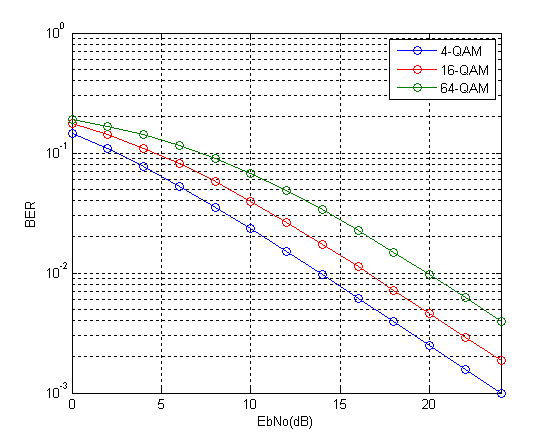So we see that the results match quite well with the results previously obtained through simulation. We will next tackle the problem of simplifying the expression for higher order modulations such as 256-QAM in both Rayleigh and Ricean channels.

# Sizing Up a Solar System for a Cellular Base Station

Many operators are thinking of moving from the main grid to alternative energy sources such as wind and solar. This is especially true in third world countries where electricity is not available 24/7 and is also very expensive. This has forced operators to switch their base stations to diesel generators (which is also a costly option).

In this article we do a rough estimation of the size a solar system required to run a cellular base station. We start with the assumption that 20 Watts of power are transmitted from a single antenna of base station. For a 3 sector site there are 3 antennas giving us total transmitted power of 60 Watts. Now if 50% of the power is lost in cables and connections we would have to boost up the transmitted power to 120 Watts.

We know that power amplifiers are highly in-efficient (depending upon the load) and a large amount of power is lost in this stage. So we assume an efficiency of 12 % giving us a total input power of 1000 Watts. Another 500 Watts are given to Air Conditioning (200 W), Signal Processing (150 W) and Rectifier (150 W). So the combined AC input to the base station is 1500 Watts. Now we turn our attention to sizing up the solar system.If we assume that the BS is continuously consuming 1500 Watts over a 24 hour period we have a total energy consumption of  36 kWh. If the solar panels receive peak sun hours of 5 hours/day we would require solar panels rated at 7200 Watts. This could mean 72 solar panels of 100 Watts each or 36 solar panels of 200 Watts each or any other combination. It must be noted that we have not considered any margins for cloudy days when peak sun hours would be reduced. Also, we have not considered any reduction in power consumption when there is no load (or very less load) on the BS.

Next we calculate the amount of batteries required. We assume that the batteries are rated at 200 AH and 12 V. This gives us a total energy storage capacity per battery of 2.4 kWh. So the number of batteries required is calculated as 36 kWh/2.4 kWh = 15. It must be noted that some of the energy would be consumed in real-time and the actual number of batteries required would be lesser. Furthermore we would need an inverter of at least 1500 Watts and charge controller of 125 Amps.

# Does Shannon Capacity Increase by Dividing a Frequency Band into Narrow Bins

Somebody recently asked me this question “Does Shannon Capacity Increase by Dividing a Frequency Band into Narrow Bins”. To be honest I was momentarily confused and thought that this may be the case since many of the modern Digital Communication Systems do use narrow frequency bins e.g. LTE. But on closer inspection I found that the Shannon Capacity does not change, in fact it remains exactly the same. Following is the reasoning for that.

Shannon Capacity is calculated as:

C=B*log2(1+SNR)

or

C=B*log2(1+P/(B*No))

Now if the bandwidth ‘B’ is divided into 10 equal blocks then the transmit power ‘P’ for each block would also be divided by 10 to keep the total transmit power for the entire band to be constant. This means that the factor P/(B*No) remains constant. So the total capacity for the 10 blocks would be calculated as:

C=10*(B/10)*log2(1+P/(B*No))

So the Shannon Capacity for the entire band remains the same.

PS: The reason for the narrower channels is that for a narrow channel the channel appears relatively flat in the frequency domain and the process of equilization is thus simplified (a simple multiplication/division would do).

Note: ‘No’ is the Noise Power Spectral Density and ‘B*No’ is the Noise Power.

# M-QAM Bit Error Rate in Rayleigh Fading

We have previously discussed the bit error rate (BER) performance of M-QAM in AWGN. We now discuss the BER performance of M-QAM in Rayleigh fading. The one-tap Rayleigh fading channel is generated from two orthogonal Gaussian random variables with variance of 0.5 each. The complex random channel coefficient so generated has an amplitude which is Rayleigh distributed and a phase which is uniformly distributed. As usual the fading channel introduces a multiplicative effect whereas the AWGN is additive.

The function “QAM_fading” has three inputs, ‘n_bits’, ‘M’, ‘EbNodB’ and one output ‘ber’. The inputs are the number of bits to be passed through the channel, the alphabet size and the Energy per Bit to Noise Power Spectral Density in dB respectively whereas the output is the bit error rate (BER).

```%%%%%%%%%%%%%%%%%%%%%%%%%%%%%%%%%%%%%%%%%%%%%%%%%%%%%%%%%%%%%%%%%%
% FUNCTION THAT CALCULATES THE BER OF M-QAM IN RAYLEIGH FADING
% n_bits: Input, number of bits
% M: Input, constellation size
% EbNodB: Input, energy per bit to noise power spectral density
% ber: Output, bit error rate
%%%%%%%%%%%%%%%%%%%%%%%%%%%%%%%%%%%%%%%%%%%%%%%%%%%%%%%%%%%%%%%%%%
% Transmitter
k=log2(M);
EbNo=10^(EbNodB/10);
x=transpose(round(rand(1,n_bits)));
h1=modem.qammod(M);
h1.inputtype='bit';
h1.symbolorder='gray';
y=modulate(h1,x);

% Channel
Eb=mean((abs(y)).^2)/k;
sigma=sqrt(Eb/(2*EbNo));
w=sigma*(randn(n_bits/k,1)+1i*randn(n_bits/k,1));
h=(1/sqrt(2))*(randn(n_bits/k,1)+1i*randn(n_bits/k,1));
r=h.*y+w;

r=r./h;
h2=modem.qamdemod(M);
h2.outputtype='bit';
h2.symbolorder='gray';
h2.decisiontype='hard decision';
z=demodulate(h2,r);
ber=(n_bits-sum(x==z))/n_bits
return
%%%%%%%%%%%%%%%%%%%%%%%%%%%%%%%%%%%%%%%%%%%%%%%%%%%%%%%%%%%%%%%%%%
```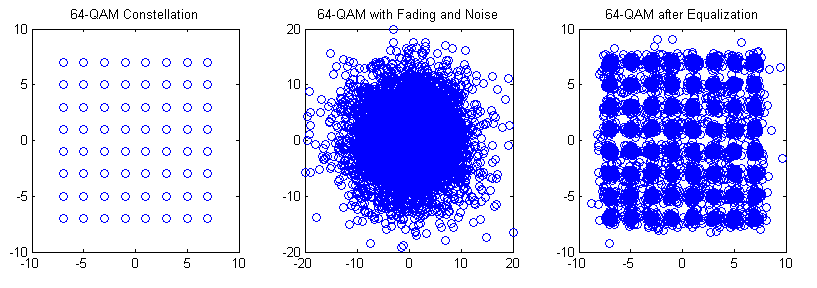The bit error rates of four modulation schemes 4-QAM, 16-QAM, 64-QAM and 256-QAM are shown in the figure above. All modulation schemes use Gray coding which gives a few dB of margin in the BER performance. As with the AWGN case each additional bit per symbol requires about 1.5-2 dB in signal to ratio to achieve the same BER.

Although not shown here similar behavior is observed for higher order modulation schemes such as 1024-QAM and 4096-QAM (the gap in the signal to noise ratio for the same BER is increased to about 5dB).

Lastly we explain some of the terms used above.